#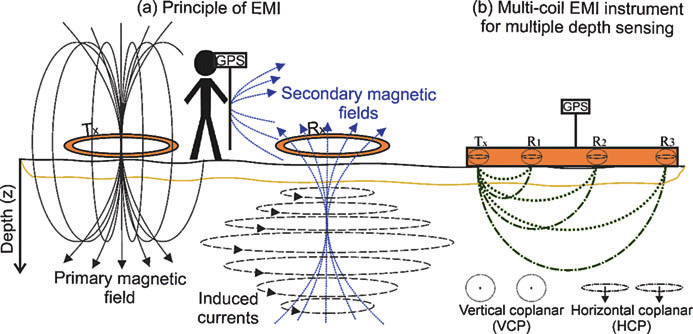Electromagnetic Induction

When a DC current passes through a long straight conductor a magnetising force and a static magnetic field is developed around it

If the wire is then wound into a coil, the magnetic field is greatly intensified producing a static magnetic field around itself forming the shape of a bar magnet giving a distinct North and South pole.

The magnetic flux developed around the coil being proportional to the amount of current flowing in the coils windings as shown. If additional layers of wire are wound upon the same coil with the same current flowing through them, the static magnetic field strength would be increased.

Therefore, the magnetic field strength of a coil is determined by the ampere turns of the coil. With more turns of wire within the coil, the greater the strength of the static magnetic field around it.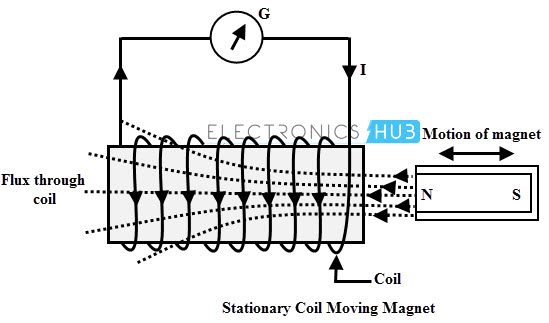But what if we reversed this idea by disconnecting the electrical current from the coil and instead of a hollow core we placed a bar magnet inside the core of the coil of wire. By moving this bar magnet “in” and “out” of the coil a current would be induced into the coil by the physical movement of the magnetic flux inside it.

Likewise, if we kept the bar magnet stationary and moved the coil back and forth within the magnetic field an electric current would be induced in the coil. Then by either moving the wire or changing the magnetic field we can induce a voltage and current within the coil and this process is known as Electromagnetic Induction and is the basic principle of operation of transformers, motors and generators.

Electromagnetic Induction was first discovered way back in the 1830’s by Michael Faraday. Faraday noticed that when he moved a permanent magnet in and out of a coil or a single loop of wire it induced an ElectroMotive Force or emf, in other words a Voltage, and therefore a current was produced.

So what Michael Faraday discovered was a way of producing an electrical current in a circuit by using only the force of a magnetic field and not batteries. This then lead to a very important law linking electricity with magnetism, Faraday’s Law of Electromagnetic Induction. So how does this work?.

When the magnet shown below is moved “towards” the coil, the pointer or needle of the Galvanometer, which is basically a very sensitive centre zero’ed moving-coil ammeter, will deflect away from its centre position in one direction only. When the magnet stops moving and is held stationary with regards to the coil the needle of the galvanometer returns back to zero as there is no physical movement of the magnetic field.

Likewise, when the magnet is moved “away” from the coil in the other direction, the needle of the galvanometer deflects in the opposite direction with regards to the first indicating a change in polarity. Then by moving the magnet back and forth towards the coil the needle of the galvanometer will deflect left or right, positive or negative, relative to the directional motion of the magnet.

### Electromagnetic Induction by a Moving Magnet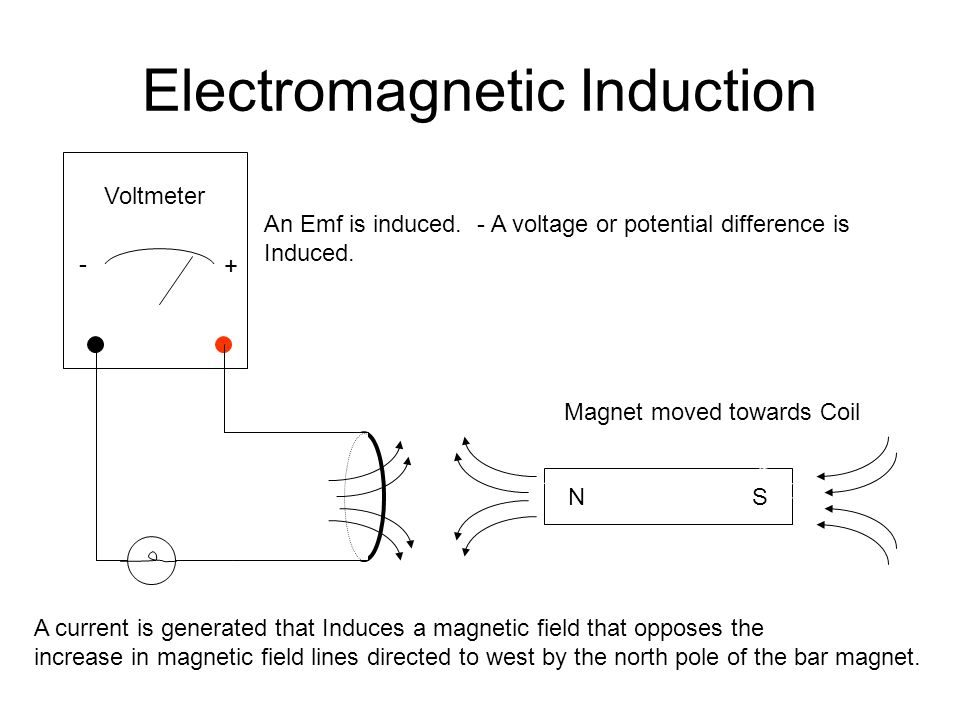Likewise, if the magnet is now held stationary and ONLY the coil is moved towards or away from the magnet the needle of the galvanometer will also deflect in either direction. Then the action of moving a coil or loop of wire through a magnetic field induces a voltage in the coil with the magnitude of this induced voltage being proportional to the speed or velocity of the movement.

Then we can see that the faster the movement of the magnetic field the greater will be the induced emf or voltage in the coil, so for Faraday’s law to hold true there must be “relative motion” or movement between the coil and the magnetic field and either the magnetic field, the coil or both can move.

From the above description we can say that a relationship exists between an electrical voltage and a changing magnetic field to which Michael Faraday’s famous law of electromagnetic induction states: “that a voltage is induced in a circuit whenever relative motion exists between a conductor and a magnetic field and that the magnitude of this voltage is proportional to the rate of change of the flux”.

In other words, Electromagnetic Induction is the process of using magnetic fields to produce voltage, and in a closed circuit, a current.

So how much voltage (emf) can be induced into the coil using just magnetism. Well this is determined by the following 3 different factors.

• 1). Increasing the number of turns of wire in the coil – By increasing the amount of individual conductors cutting through the magnetic field, the amount of induced emf produced will be the sum of all the individual loops of the coil, so if there are 20 turns in the coil there will be 20 times more induced emf than in one piece of wire.
• 2). Increasing the speed of the relative motion between the coil and the magnet – If the same coil of wire passed through the same magnetic field but its speed or velocity is increased, the wire will cut the lines of flux at a faster rate so more induced emf would be produced.
• 3). Increasing the strength of the magnetic field – If the same coil of wire is moved at the same speed through a stronger magnetic field, there will be more emf produced because there are more lines of force to cut.

If we were able to move the magnet in the diagram above in and out of the coil at a constant speed and distance without stopping we would generate a continuously induced voltage that would alternate between one positive polarity and a negative polarity producing an alternating or AC output voltage and this is the basic principle of how an electrical generator works similar to those used in dynamos and car alternators.

In small generators such as a bicycle dynamo, a small permanent magnet is rotated by the action of the bicycle wheel inside a fixed coil. Alternatively, an electromagnet powered by a fixed DC voltage can be made to rotate inside a fixed coil, such as in large power generators producing in both cases an alternating current.

### Simple Generator using Magnetic Induction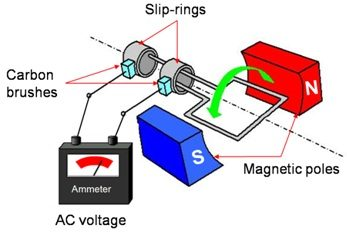The simple dynamo type generator above consists of a permanent magnet which rotates around a central shaft with a coil of wire placed next to this rotating magnetic field. As the magnet spins, the magnetic field around the top and bottom of the coil constantly changes between a north and a south pole. This rotational movement of the magnetic field results in an alternating emf being induced into the coil as defined by Faraday’s law of electromagnetic induction.

The magnitude of the electromagnetic induction is directly proportional to the flux density, β the number of loops giving a total length of the conductor, l in meters and the rate or velocity, ν at which the magnetic field changes within the conductor in meters/second or m/s, giving by the motional emf expression: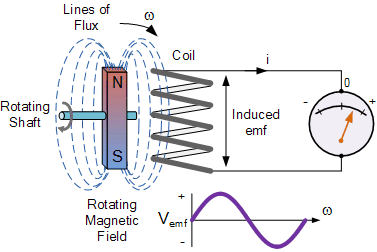If the conductor does not move at right angles (90°) to the magnetic field then the angle θ° will be added to the above expression giving a reduced output as the angle increases:

## Lenz’s Law of Electromagnetic Induction

Faraday’s Law tells us that inducing a voltage into a conductor can be done by either passing it through a magnetic field, or by moving the magnetic field past the conductor and that if this conductor is part of a closed circuit, an electric current will flow. This voltage is called an induced emf as it has been induced into the conductor by a changing magnetic field due to electromagnetic induction with the negative sign in Faraday’s law telling us the direction of the induced current (or polarity of the induced emf).

But a changing magnetic flux produces a varying current through the coil which itself will produce its own magnetic field as we saw in the Electromagnets tutorial. This self-induced emf opposes the change that is causing it and the faster the rate of change of current the greater is the opposing emf. This self-induced emf will, by Lenz’s law oppose the change in current in the coil and because of its direction this self-induced emf is generally called a back-emf.

Lenz’s Law states that: ” the direction of an induced emf is such that it will always opposes the change that is causing it”. In other words, an induced current will always OPPOSE the motion or change which started the induced current in the first place and this idea is found in the analysis of Inductance.

Likewise, if the magnetic flux is decreased then the induced emf will oppose this decrease by generating and induced magnetic flux that adds to the original flux.

Lenz’s law is one of the basic laws in electromagnetic induction for determining the direction of flow of induced currents and is related to the law of conservation of energy.

According to the law of conservation of energy which states that the total amount of energy in the universe will always remain constant as energy can not be created nor destroyed. Lenz’s law is derived from Michael Faraday’s law of induction.

One final comment about Lenz’s Law regarding electromagnetic induction. We now know that when a relative motion exists between a conductor and a magnetic field, an emf is induced within the conductor.

But the conductor may not actually be part of the coils electrical circuit, but may be the coils iron core or some other metallic part of the system, for example, a transformer. The induced emf within this metallic part of the system causes a circulating current to flow around it and this type of core current is known as an Eddy Current.

Eddy currents generated by electromagnetic induction circulate around the coils core or any connecting metallic components inside the magnetic field because for the magnetic flux they are acting like a single loop of wire. Eddy currents do not contribute anything towards the usefulness of the system but instead they oppose the flow of the induced current by acting like a negative force generating resistive heating and power loss within the core. However, there are electromagnetic induction furnace applications in which only eddy currents are used to heat and melt ferromagnetic metals.

### Eddy Currents Circulating in a Transformer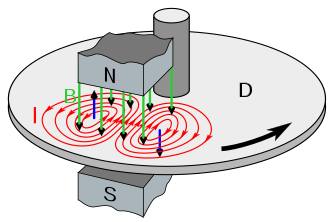The changing magnetic flux in the iron core of a transformer above will induce an emf, not only in the primary and secondary windings, but also in the iron core. The iron core is a good conductor, so the currents induced in a solid iron core will be large. Furthermore, the eddy currents flow in a direction which, by Lenz’s law, acts to weaken the flux created by the primary coil. Consequently, the current in the primary coil required to produce a given B field is increased, so the hysteresis curves are fatter along the H axis.

Eddy current and hysteresis losses can not be eliminated completely, but they can be greatly reduced. Instead of having a solid iron core as the magnetic core material of the transformer or coil, the magnetic path is “laminated”.

These laminations are very thin strips of insulated (usually with varnish) metal joined together to produce a solid core. The laminations increase the resistance of the iron-core thereby increasing the overall resistance to the flow of the eddy currents, so the induced eddy current power-loss in the core is reduced, and it is for this reason why the magnetic iron circuit of transformers and electrical machines are all laminated.

## STEM Elearning

We at FAWE have built this platform to aid learners, trainers and mentors get practical help with content, an interactive platform and tools to power their teaching and learning of STEM subjects, more